# Contexts¶

High-level code in mpmath is implemented as methods on a “context object”. The context implements arithmetic, type conversions and other fundamental operations. The context also holds settings such as precision, and stores cache data. A few different contexts (with a mostly compatible interface) are provided so that the high-level algorithms can be used with different implementations of the underlying arithmetic, allowing different features and speed-accuracy tradeoffs. Currently, mpmath provides the following contexts:

• Arbitrary-precision arithmetic (mp)
• A faster Cython-based version of mp (used by default in Sage, and currently only available there)
• Arbitrary-precision interval arithmetic (iv)
• Double-precision arithmetic using Python’s builtin float and complex types (fp)

Most global functions in the global mpmath namespace are actually methods of the mp context. This fact is usually transparent to the user, but sometimes shows up in the form of an initial parameter called “ctx” visible in the help for the function:

>>> import mpmath
>>> help(mpmath.fsum)
Help on method fsum in module mpmath.ctx_mp_python:

fsum(ctx, terms, absolute=False, squared=False) method of mpmath.ctx_mp.MPContext instance
Calculates a sum containing a finite number of terms (for infinite
series, see :func:~mpmath.nsum). The terms will be converted to
...


The following operations are equivalent:

>>> mpmath.mp.dps = 15; mpmath.mp.pretty = False
>>> mpmath.fsum([1,2,3])
mpf('6.0')
>>> mpmath.mp.fsum([1,2,3])
mpf('6.0')


The corresponding operation using the fp context:

>>> mpmath.fp.fsum([1,2,3])
6.0


## Common interface¶

ctx.mpf creates a real number:

>>> from mpmath import mp, fp
>>> mp.mpf(3)
mpf('3.0')
>>> fp.mpf(3)
3.0


ctx.mpc creates a complex number:

>>> mp.mpc(2,3)
mpc(real='2.0', imag='3.0')
>>> fp.mpc(2,3)
(2+3j)


ctx.matrix creates a matrix:

>>> mp.matrix([[1,0],[0,1]])
matrix(
[['1.0', '0.0'],
['0.0', '1.0']])
>>> _[0,0]
mpf('1.0')
>>> fp.matrix([[1,0],[0,1]])
matrix(
[['1.0', '0.0'],
['0.0', '1.0']])
>>> _[0,0]
1.0


ctx.prec holds the current precision (in bits):

>>> mp.prec
53
>>> fp.prec
53


ctx.dps holds the current precision (in digits):

>>> mp.dps
15
>>> fp.dps
15


ctx.pretty controls whether objects should be pretty-printed automatically by repr(). Pretty-printing for mp numbers is disabled by default so that they can clearly be distinguished from Python numbers and so that eval(repr(x)) == x works:

>>> mp.mpf(3)
mpf('3.0')
>>> mpf = mp.mpf
>>> eval(repr(mp.mpf(3)))
mpf('3.0')
>>> mp.pretty = True
>>> mp.mpf(3)
3.0
>>> fp.matrix([[1,0],[0,1]])
matrix(
[['1.0', '0.0'],
['0.0', '1.0']])
>>> fp.pretty = True
>>> fp.matrix([[1,0],[0,1]])
[1.0  0.0]
[0.0  1.0]
>>> fp.pretty = False
>>> mp.pretty = False


## Arbitrary-precision floating-point (mp)¶

The mp context is what most users probably want to use most of the time, as it supports the most functions, is most well-tested, and is implemented with a high level of optimization. Nearly all examples in this documentation use mp functions.

See Basic usage for a description of basic usage.

## Arbitrary-precision interval arithmetic (iv)¶

The iv.mpf type represents a closed interval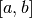; that is, the set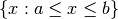, where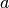and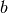are arbitrary-precision floating-point values, possibly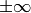. The iv.mpc type represents a rectangular complex interval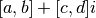; that is, the set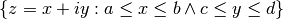.

Interval arithmetic provides rigorous error tracking. If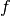is a mathematical function and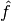is its interval arithmetic version, then the basic guarantee of interval arithmetic is that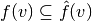for any input interval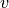. Put differently, if an interval represents the known uncertainty for a fixed number, any sequence of interval operations will produce an interval that contains what would be the result of applying the same sequence of operations to the exact number. The principal drawbacks of interval arithmetic are speed (iv arithmetic is typically at least two times slower than mp arithmetic) and that it sometimes provides far too pessimistic bounds.

Note

The support for interval arithmetic in mpmath is still experimental, and many functions do not yet properly support intervals. Please use this feature with caution.

Intervals can be created from single numbers (treated as zero-width intervals) or pairs of endpoint numbers. Strings are treated as exact decimal numbers. Note that a Python float like 0.1 generally does not represent the same number as its literal; use '0.1' instead:

>>> from mpmath import iv
>>> iv.dps = 15; iv.pretty = False
>>> iv.mpf(3)
mpi('3.0', '3.0')
>>> print iv.mpf(3)
[3.0, 3.0]
>>> iv.pretty = True
>>> iv.mpf([2,3])
[2.0, 3.0]
>>> iv.mpf(0.1)   # probably not intended
[0.10000000000000000555, 0.10000000000000000555]
>>> iv.mpf('0.1')   # good, gives a containing interval
[0.099999999999999991673, 0.10000000000000000555]
>>> iv.mpf(['0.1', '0.2'])
[0.099999999999999991673, 0.2000000000000000111]


The fact that '0.1' results in an interval of nonzero width indicates that 1/10 cannot be represented using binary floating-point numbers at this precision level (in fact, it cannot be represented exactly at any precision).

Intervals may be infinite or half-infinite:

>>> print 1 / iv.mpf([2, 'inf'])
[0.0, 0.5]


The equality testing operators == and != check whether their operands are identical as intervals; that is, have the same endpoints. The ordering operators < <= > >= permit inequality testing using triple-valued logic: a guaranteed inequality returns True or False while an indeterminate inequality returns None:

>>> iv.mpf([1,2]) == iv.mpf([1,2])
True
>>> iv.mpf([1,2]) != iv.mpf([1,2])
False
>>> iv.mpf([1,2]) <= 2
True
>>> iv.mpf([1,2]) > 0
True
>>> iv.mpf([1,2]) < 1
False
>>> iv.mpf([1,2]) < 2    # returns None
>>> iv.mpf([2,2]) < 2
False
>>> iv.mpf([1,2]) <= iv.mpf([2,3])
True
>>> iv.mpf([1,2]) < iv.mpf([2,3])  # returns None
>>> iv.mpf([1,2]) < iv.mpf([-1,0])
False


The in operator tests whether a number or interval is contained in another interval:

>>> iv.mpf([0,2]) in iv.mpf([0,10])
True
>>> 3 in iv.mpf(['-inf', 0])
False


Intervals have the properties .a, .b (endpoints), .mid, and .delta (width):

>>> x = iv.mpf([2, 5])
>>> x.a
[2.0, 2.0]
>>> x.b
[5.0, 5.0]
>>> x.mid
[3.5, 3.5]
>>> x.delta
[3.0, 3.0]


Some transcendental functions are supported:

>>> iv.dps = 15
>>> mp.dps = 15
>>> iv.mpf([0.5,1.5]) ** iv.mpf([0.5, 1.5])
[0.35355339059327373086, 1.837117307087383633]
>>> iv.exp(0)
[1.0, 1.0]
>>> iv.exp(['-inf','inf'])
[0.0, +inf]
>>>
>>> iv.exp(['-inf',0])
[0.0, 1.0]
>>> iv.exp([0,'inf'])
[1.0, +inf]
>>> iv.exp([0,1])
[1.0, 2.7182818284590455349]
>>>
>>> iv.log(1)
[0.0, 0.0]
>>> iv.log([0,1])
[-inf, 0.0]
>>> iv.log([0,'inf'])
[-inf, +inf]
>>> iv.log(2)
[0.69314718055994528623, 0.69314718055994539725]
>>>
>>> iv.sin([100,'inf'])
[-1.0, 1.0]
>>> iv.cos(['-0.1','0.1'])
[0.99500416527802570954, 1.0]


Interval arithmetic is useful for proving inequalities involving irrational numbers. Naive use of mp arithmetic may result in wrong conclusions, such as the following:

>>> mp.dps = 25
>>> x = mp.exp(mp.pi*mp.sqrt(163))
>>> y = mp.mpf(640320**3+744)
>>> print x
262537412640768744.0000001
>>> print y
262537412640768744.0
>>> x > y
True


But the correct result is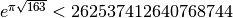, as can be seen by increasing the precision:

>>> mp.dps = 50
>>> print mp.exp(mp.pi*mp.sqrt(163))
262537412640768743.99999999999925007259719818568888


With interval arithmetic, the comparison returns None until the precision is large enough for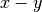to have a definite sign:

>>> iv.dps = 15
>>> iv.exp(iv.pi*iv.sqrt(163)) > (640320**3+744)
>>> iv.dps = 30
>>> iv.exp(iv.pi*iv.sqrt(163)) > (640320**3+744)
>>> iv.dps = 60
>>> iv.exp(iv.pi*iv.sqrt(163)) > (640320**3+744)
False
>>> iv.dps = 15


## Fast low-precision arithmetic (fp)¶

Although mpmath is generally designed for arbitrary-precision arithmetic, many of the high-level algorithms work perfectly well with ordinary Python float and complex numbers, which use hardware double precision (on most systems, this corresponds to 53 bits of precision). Whereas the global functions (which are methods of the mp object) always convert inputs to mpmath numbers, the fp object instead converts them to float or complex, and in some cases employs basic functions optimized for double precision. When large amounts of function evaluations (numerical integration, plotting, etc) are required, and when fp arithmetic provides sufficient accuracy, this can give a significant speedup over mp arithmetic.

To take advantage of this feature, simply use the fp prefix, i.e. write fp.func instead of func or mp.func:

>>> u = fp.erfc(2.5)
>>> print u
0.000406952017445
>>> type(u)
<type 'float'>
>>> mp.dps = 15
>>> print mp.erfc(2.5)
0.000406952017444959
>>> fp.matrix([[1,2],[3,4]]) ** 2
matrix(
[['7.0', '10.0'],
['15.0', '22.0']])
>>>
>>> type(_[0,0])
<type 'float'>
>>> print fp.quad(fp.sin, [0, fp.pi])    # numerical integration
2.0


The fp context wraps Python’s math and cmath modules for elementary functions. It supports both real and complex numbers and automatically generates complex results for real inputs (math raises an exception):

>>> fp.sqrt(5)
2.23606797749979
>>> fp.sqrt(-5)
2.23606797749979j
>>> fp.sin(10)
-0.5440211108893698
>>> fp.power(-1, 0.25)
(0.7071067811865476+0.7071067811865475j)
>>> (-1) ** 0.25
Traceback (most recent call last):
...
ValueError: negative number cannot be raised to a fractional power


The prec and dps attributes can be changed (for interface compatibility with the mp context) but this has no effect:

>>> fp.prec
53
>>> fp.dps
15
>>> fp.prec = 80
>>> fp.prec
53
>>> fp.dps
15


Due to intermediate rounding and cancellation errors, results computed with fp arithmetic may be much less accurate than those computed with mp using an equivalent precision (mp.prec = 53), since the latter often uses increased internal precision. The accuracy is highly problem-dependent: for some functions, fp almost always gives 14-15 correct digits; for others, results can be accurate to only 2-3 digits or even completely wrong. The recommended use for fp is therefore to speed up large-scale computations where accuracy can be verified in advance on a subset of the input set, or where results can be verified afterwards.